以纯小白的角度看 动态规划（DP学习总结）

引言

1+1+1+1+1+1+1+1=?

斐波那契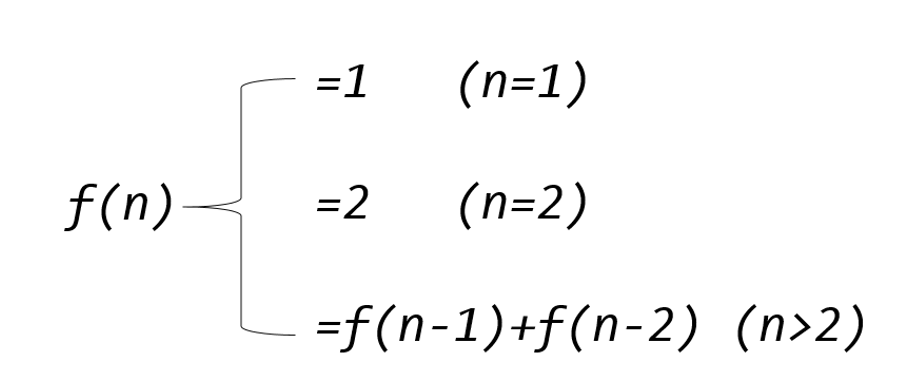int fib(int n)
{
if(n<=1)
return 1;
else
return fib( n-1)+fib(n-2);
}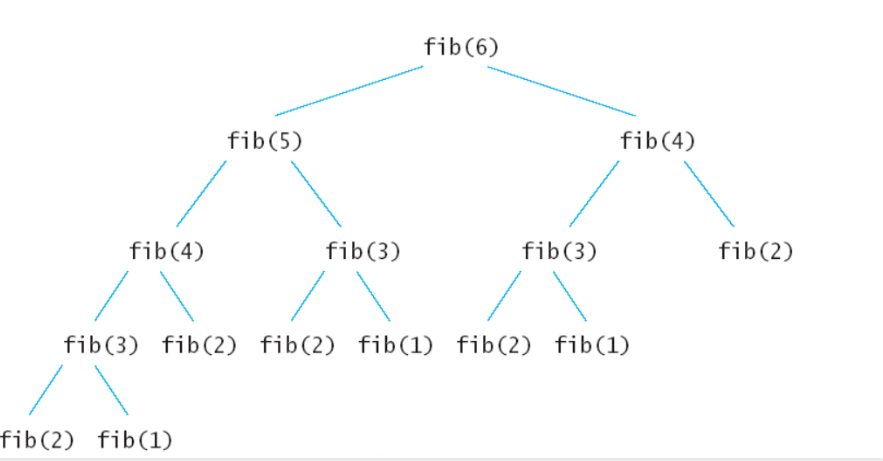#define Max 1000
int memory[Max]={0};
//  备忘录
int fib(n)
{
if(memory[n]!=0)
return memory[n];
//表中已有，直接返回
//  否则，写入备忘录
if(n<=1)
memory[n]=1;
else
memory[n]=fib(n-1)+fib(n-2);

return memory[n];
}

#define Max 1000
int tab[Max]={0};
// 表
int fib(n)
{
tab=tab=1
for(int i=2;i<=n;i++)
tab[i]=tab[i-1]+tab[i-2];

return tab[n];
}

1.切原木问题–线性模型：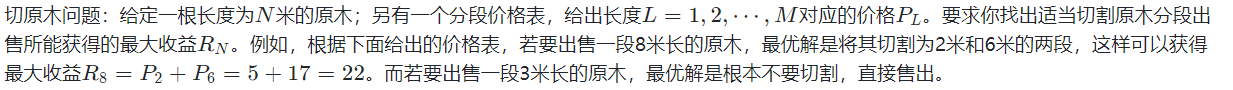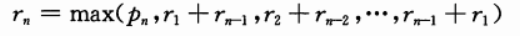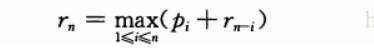#define Max 1000
#define Min_value -1
int p[Max];
//价格表
int max(int a,int b)
{
return a>b?a:b;
}
int cut(n)
{
if(n==0)
return 0;
if(n==1)
return 1;

int q=Min_value;
//最大价格
for(int i=1;i<=n;i++)
{
q=max(q,p[i-1]+cut(n-i));
}

return q;
}

#define Max 1000
#define Min_value -1
int p[Max];
//价格表
int memory[Max]={0};
//备忘录
int max(int a,int b)
{
return a>b?a:b;
}
int cut(n)
{
if(memory[n]!=0)
return memory[n];

if(n==1)
memory[n]=p;
else
{
int q=Min_value;
//最大价格
for(int i=1;i<=n;i++)
{
q=max(q,p[i-1]+cut(n-i));
}
memory[n]=q;
}

return memory[n];
}

#define Max 1000
#define Min_value -1
int p[Max];
//价格表
int r[Max];
//最优解表
int max(int a,int b)
{
return a>b?a:b;
}
int cut(n)
{
if(n<=0)return 0;

for(int i=1;i<=n;i++)
{
int q=Min_value;
//自底向上动态规划的精髓
for(int j=1;j<=i;j++)
{
q=max(q,p[j]+cut[i-j]);
}
r[i]=q;
}

return r[n];
}

1.划分子问题：

2.确定状态

3.确定一些初始状态（边界状态）的值

4. 确定状态转移方程

2.构造回文串问题–区间模型

1、在A[j]后面添加一个字符A[i]；
2、在A[i]前面添加一个字符A[j]；

d[i][j] = min{ d[i+1][j], d[i][j-1] } + 1

#define Max 1000
char temp[Max];
int d[Max][Max]={0};
int min(int a,int b)
{
return a<b?a:b;
}
int minPut(int i,int j)
{
if(d[i][j]!=0)return d[i][j];//查到

if(i>j)return 0;//空串

if(temp[i]==temp[j])
d[i][j]=minPut(i+1,j-1);
else
d[i][j]=min(minPut(i+1,j),minPut(i,j-1))+1;

return d[i][j];
}

#define Max 1000
char temp[Max];
int d[Max][Max]={0};
int min(int a,int b)
{
return a<b?a:b;
}
int minPut(int i,int j)
{
for(int k=1;k<=j-i;k++)
{
//不同阶段
for(int q=i;q<=j-k;q++)
{
//求该阶段的状态
if(temp[q]==temp[q+k])
{
d[q][q+k]=d[q+1][q+k-1];
}
else
{
d[q][q+k]=min(d[q+1][q+k],d[q][q+k-1])+1;
}
}
}
return d[i][j];
}

3.背包模型

f[i][v] = max{ f[i-1][v], f[i-1][v – Ci] +Pi }

int n=5;//数量
int bag=10;//容量
int v={0,2,2,6,5,4};//体积
int p={0,6,3,5,4,6};//价值
int f={0};//状态

int max(int a,int b)
{
return a>b?a:b;
}

int take()
{
for(int i=1;i<=n;i++)
{
for(int j=1;j<=bag;j++)
{
if(j>=p[i])
f[i][j]=max(f[i-1][j],f[i-1][j-v[i]]+p[i]);
else
f[i][j]=f[i-1][j];
}
}
return f[n][bag];
}

(1) 最优子结构：如果问题的最优解所包含的子问题的解也是最优的，就称该问题具有最优子结构，即满足最优化原理。

(2) 无后效性：即某阶段状态一旦确定，就不受这个状态以后决策的影响。也就是说，某状态以后的过程不会影响以前的状态，只与当前状态有关。

（3）有重叠子问题：即子问题之间是不独立的，一个子问题在下一阶段决策中可能被多次使用到。（不是动态规划施展的必要条件，但是一旦问题中有重叠子问题存在，动态规划往往比别的算法有更好的效益）

各算法之间的比较

1.相较于贪心法：

1）当n=1时,问题成立.
2）假设当n=i 时问题成立,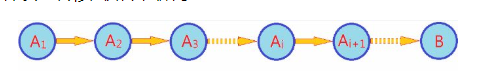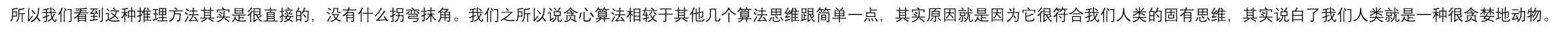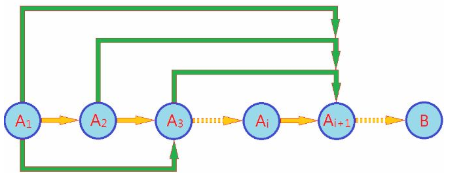{A1->A2}; {A1, A2->A3}; {A1,A2,A3->A4};……; {A1,A2,…,Ai}->Ai+1. 即对于Ai+1需要前面的所有前序状态才能完成推理过程。这种方式就是第二数学归纳法，我们将这一模型称为高阶马尔科夫模型。对应的推理过程叫做“动态规划法”

2.相较于分而治之

int Max3( int A, int B, int C )
{ /* 返回3个整数中的最大值 */
return A > B ? A > C ? A : C : B > C ? B : C;
}
int DivideAndConquer( int List[], int left, int right )
{ /* 分治法求List[left]到List[right]的最大子列和 */
int MaxLeftSum, MaxRightSum; /* 存放左右子问题的解 */
int MaxLeftBorderSum, MaxRightBorderSum; /*存放跨分界线的结果*/

int LeftBorderSum, RightBorderSum;
int center, i;

if( left == right )  { /* 递归的终止条件，子列只有1个数字 */
if( List[left] > 0 )  return List[left];
else return 0;
}

/* 下面是"分"的过程 */
center = ( left + right ) / 2; /* 找到中分点 */
/* 递归求得两边子列的最大和 */
MaxLeftSum = DivideAndConquer( List, left, center );
MaxRightSum = DivideAndConquer( List, center+1, right );

/* 下面求跨分界线的最大子列和 */
MaxLeftBorderSum = 0; LeftBorderSum = 0;
for( i=center; i>=left; i-- ) { /* 从中线向左扫描 */
LeftBorderSum += List[i];
if( LeftBorderSum > MaxLeftBorderSum )
MaxLeftBorderSum = LeftBorderSum;
} /* 左边扫描结束 */

MaxRightBorderSum = 0; RightBorderSum = 0;
for( i=center+1; i<=right; i++ ) { /* 从中线向右扫描 */
RightBorderSum += List[i];
if( RightBorderSum > MaxRightBorderSum )
MaxRightBorderSum = RightBorderSum;
} /* 右边扫描结束 */

/* 下面返回"治"的结果 */
return Max3( MaxLeftSum, MaxRightSum, MaxLeftBorderSum + MaxRightBorderSum );
}

r[i]=max(A[i],r[i-1]+A[i]);

#define Max 1000
#define Min -1000
int A[Max]={Min,-2,11,-4,13,-5,-2};
int r[Max];//表示以A[i]为末尾的连续序列的最大和(必须以A[i]为结尾)
int max(int a,int b)
{
return a>b?a:b;
}
int DP(int n)
{
int ans=Min;
for(int i=1;i<=n;i++)
{
r[i]=max(A[i],r[i-1]+A[i]);
//在所有以A[i]结尾的连续最大序列和中找出最大的r[i]
ans=max(ans,r[i]);
}
return ans;
}

3.相较于回溯剪枝

4.总结如下：

参考博客：

07-074044
01-24750208-303691
09-2547
07-1531万+
11-23280
08-212943
08-11104
03-251093
09-192394
07-245015
03-311643
07-291044
10-302062
02-171816
12-12754
03-30326
11-03791
10-293136
03-14215Reza.

¥2 ¥4 ¥6 ¥10 ¥20余额支付 (余额：-- )扫码支付获取中扫码支付点击重新获取扫码支付1.余额是钱包充值的虚拟货币，按照1:1的比例进行支付金额的抵扣。
2.余额无法直接购买下载，可以购买VIP、C币套餐、付费专栏及课程。余额充值